NEET  >  DC Pandey Solutions: Thermometry, Thermal Expansion & Kinetic Theory of Gases - 1

# DC Pandey Solutions: Thermometry, Thermal Expansion & Kinetic Theory of Gases - 1 - Notes | Study Physics Class 11 - NEET

 1 Crore+ students have signed up on EduRev. Have you?

Introductory Exercise 17.1

Ques 1: What is the value of
(a) 0°F in celsius scale?
(b) 0 K on Fahrenheit scale?
Sol: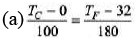Putting TF = 0, we get TC = - 17.8°C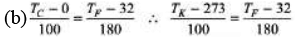Ques 2: At what temperature is the Fahrenheit scale reading equal to (a) twice (b) half of Celsius?
Sol: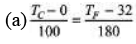Putting TF = 2Twe get TC = 160° C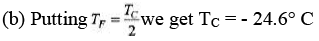Ques 3: A Fault y thermometer reads 5°C in melting ice and 99°C in steam. Find the correct temperature in °F when this faulty thermometer reads 52°C.
Sol: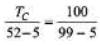∴ TC = 50°c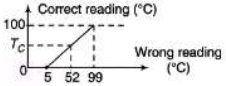Now using the equation,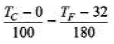Putting TC = 50° C, we get TF = 122°F

Ques 4: At what temperature the Fahrenheit and Kelvin scales of temperature give the same reading?
Sol: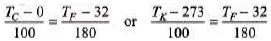Putting TF = TK,
We get, TF or TK = 574.25

Ques 5: At what temperature the Fahrenheit and Celsius scales of temperature give the same reading?
Sol: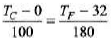Putting TC =TF, we get
TC or TF =-40°C or -40°F

Ques 6: A pendulum clock of time period 2 sec gives the correct time at 30°C. The pendulum is made of iron. How many seconds will it lose or gain per day when the temperature falls to 0°C?
αFe = 1.2 × 10-5 (°C)-1.
Sol: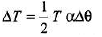Temperature is decreased. Hence l or T will decrease. So it will gain time.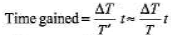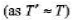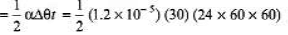= 15.55 s

Ques 7: A block of wood is floating in water at 0°C. The temperature of water is slowly raised from 0°C to 10°C. How will the percentage of volume of block above water level change with rise in temperature?
Sol:
Density of water will increase by increasing the temperature from 0 to 4°C, then it will decrease. Fraction of volume immersed is given by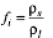ρ1 is first increased the decreased first decreased the increased.
Or percentage of volume above water level will first increased then decreased.

Ques 8: A piece of metal floats on mercury. The coefficient of volume expansion of metal and mercury are γ1 and γ2 respectively. If the temperature of both mercury and metal are increased by an amount ΔT, by what factor does the fraction of the volume of the metal submerged in mercury changes?
Sol: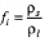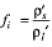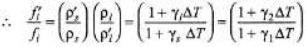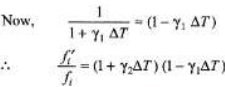Neglecting γ1γ2(DT)2 term, we get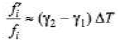Ques 9: A brass disc fit s snugly in a hole in a steel plate. Should you heat or cool the system to loosen the disc from the hole? Given that αB > aFe.
Sol: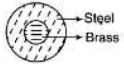Since, αB >αFe
On cooling brass will contract more.

Ques 10:Show that the volume thermal expansion coefficient for an ideal gas at constant pressure is 1/T.
Sol:
PV = nRT ...(i)
At constant pressure we can write the above equation as
PdV = nRdT .
∴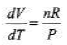...(ii)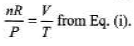Substituting in Eq. (ii),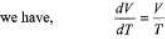or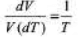...(iii)
Now we have an equation,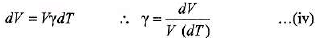From Eqs. (iii) and (iv), we get, γ= 1/T

The document DC Pandey Solutions: Thermometry, Thermal Expansion & Kinetic Theory of Gases - 1 - Notes | Study Physics Class 11 - NEET is a part of the NEET Course Physics Class 11.
All you need of NEET at this link: NEET

## Physics Class 11

127 videos|464 docs|210 tests

## Physics Class 11

127 videos|464 docs|210 tests

### How to Prepare for NEET

Read our guide to prepare for NEET which is created by Toppers & the best Teachers

Track your progress, build streaks, highlight & save important lessons and more!

,

,

,

,

,

,

,

,

,

,

,

,

,

,

,

,

,

,

,

,

,

,

,

,

;